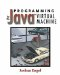# 5.3 Other Comparisons

All control instructions test either ints or references. If you want to do a branch based on a comparison between two floats, longs, or doubles, you have to use a separate instruction to turn the non-int comparison into an int comparison. The instructions to do this are summarized in Table 5.2. These instructions leave 1, 0, or 1 on the stack if the first operand is greater than, equal to, or less than the second. This value is easily compared with zero as an int, using the ifeq, ifne, iflt, ifgt, ifle, or ifge instructions. For example, comparing double values:

` getstatic java/lang/Math/PI D  ; Get a good approximation of pi ldc2_w 3.1416D                 ; Get a worse approximation of pi dcmpg                          ; Compare the two numbers                                ; This leaves  1 on the stack,                                ; since pi < 3.1416 iflt approxTooHigh             ; Goes to approxTooHigh, since                                ;  1 < 0 `

##### Table 5.2. Comparison operations
Mnemonic Stack Effect
dcmpg double1 double2

If double1 == double2, leave 0 on the stack.

If double1 < double1, leave 1.

If double1 > double1, leave 1.

If either is NaN, leave 1.

dcmpl double1 double2 Same as dcmpg, except leave 1 if either is NaN.
fcmpg float1 float2

If float1 == float2, leave 0 on the stack.

If float1 < float2, leave 1.

If float1 > float2, leave 1.

If either is NaN, leave 1.

fcmpl float1 float2 Same as fcmpg,"except leave 1 if either is NaN.
lcmp long1 long2

If long1 == long2, leave 0 on the stack.

If long1 < long2, leave 1.

If long1 > long2, leave 1.

Or for long values:

` ; Push the current time in milliseconds, a long value invokestatic java/lang/System/currentTimeMillis()J aload_0                   ; Push a Document getfield Document/lastUpdatedTime J                           ; Get the last time this                           ; document was updated lsub                      ; Figure out the difference ldc 60000                 ; Was it more than 60 seconds ago? lcmp                      ; Leaves 1 if more than 60 seconds                           ; 0 if exactly 60 seconds, and                           ;  1 if less than 60 seconds ifle makeBackup           ; Make a backup if it was updated                           ; <= 60 seconds ago `

For the float and double comparisons, there are two almost identical operations for each: fcmpg/fcmpl and dcmpg/dcmpl. The difference is in the treatment of values that are NaN (not a number). Since NaN isn't really a number, it can't be compared to the other values. You choose the g variant or the l variant based on how you want your program to react if it encounters invalid numbers. If you want to see if a > b and you use dcmpg, then the test will succeed if either is NaN. If you use dcmpl, it will to fail.Programming for the Javaв„ў Virtual Machine
ISBN: 0201309726
EAN: 2147483647
Year: 1998
Pages: 158
Authors: Joshua Engel

Similar book on Amazon